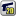﻿ Unreal Software - Thread: Round off decimal value

# ForumCS2DScriptsRound off decimal value

#Round off decimal value

7 replies
Goto Page1Ace Howl
User
OfflineHello, currently I'm inactive playing this game (sad much) but I have a 'thing' that need to be solved.

As stated from the title, I searched for round off, decimal and whole but found none. So, the question is: how to round off decimal value into whole number.

For example, using 'distance between 2 players' code (distance between two points [Pythagoras Theorem thing]) code will return long-ass decimal values (e.g. 3.69420666) most of the instance. That is inconvenient for me during actual gameplay.

Any ideas?
Starkkz
Moderator
OfflineSome people uses
math.floor(x + 0.5)
.
Baloon
GAME BANNED
OfflineUse this math. calculation
math.floor
or
math.ceil

Example:
Code:
1
2
3
4
5
6
7
math.floor(6.12314) > 6
math.floor(7.90999) > 7
math.floor(3)          > 3

math.ceil(1.00001) > 2
math.ceil(5.93213) > 6
math.ceil(2)          > 2

Zeik
User
OfflineAsStarkkz said, use
math.floor(x + 0.5)
whether you want to round up if the fractional part of x is greater or equal than 0.5, or whether you want to round down if the fractional part of x is lower than 0.5.
Note that it would only work with positive numbers, as it would be the opposite for negative numbers to work:
math.floor(x - 0.5)
.

If you just want to round down no matter what is the fractional part, just use
math.floor(x)
Ace Howl
User
OfflineAnd how does that operation work?
Let's say I've calculated some Pythagoras value and is in fraction.
Code:
1
2
3
4
5
-- As example only
local neardist = (contain-decimal-value)
if txt==("nearest") then
parse("msg2 "..id.." "..neardist)
end

Where should I settle the job?
Baloon
GAME BANNED
OfflineIf that's you want (distance between- ) i can't do that, in my way, that needs much math codes like math.rad, math.cos, math.tan, etc.
Rainoth
Moderator
Offline@Ace Howl: So what don't you get? It rounds the number. You can't add fractions in your head and see how it works..? I don't get you.

Here's a few example of what
math.floor(x + 0.5)
does when x is:
0.512341
0.4987650
420.69421
419.50420
777.321777

Just take whatever number and whatever fraction it has after you add 0.5 you take away.

@Baloon: AsAce Howl said, the only thing you need to get distance is basic understanding of pythagorean theorem. They teach those in 5th-6th grade I guess, maybe earlier.
MALDeviantArtFor Wallpaper Engine usersEngiN33R
Moderator
OfflineOn its own, the function
math.floor(a)
finds such integer n (n ∈ Z) that n ≤ a. Thus, for any positive number it will return that number without its fractional part - 0.3 becomes 0, 1.8 becomes 1, 17.5 becomes 17 etc. For any negative number, it will return the number before it, so -1.3 becomes -2, -4.75 becomes -5 etc.

The trick behind
math.floor(a+0.5)
is that any number with a fractional part smaller than 0.5 retains the same integer part (0.3 + 0.5 is 0.8, the integer part is still 0), whereas for any number with a fractional part larger than or equal to 0.5 the integer part will increase by 1, and the fractional part will be removed (0.75 + 0.5 is 1.25, or 1 without the fractional part thanks to flooring).

Hence, you should use this whenever you want your value to be rounded. That depends entirely on you. In your sample code, you can do the following:
Code:
1
2
3
4
local neardist = math.floor(math.sqrt((x-x2)^2+(y-y2)^2)+0.5)
if (txt == "nearest") then
msg2(id, neardist)
end

Alternatively, if you want to store the exact distance and only display it in a user-friendly way, you can do it like this:
Code:
1
2
3
4
local neardist = math.sqrt((x-x2)^2+(y-y2)^2)
if (txt == "nearest") then
msg2(id, math.floor(neardist+0.5))
end
I code, therefore I exist. | Visit my blog for Lua tips and other interesting info1﻿